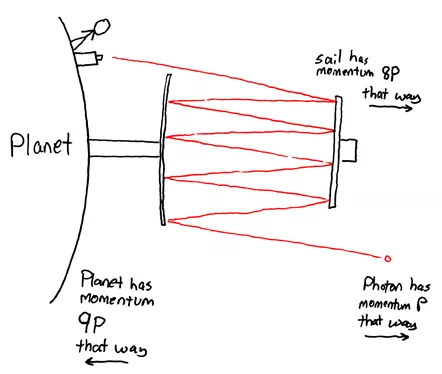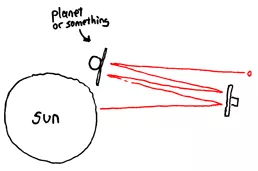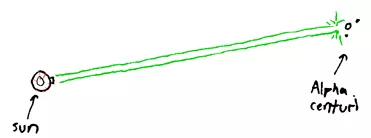# 4.6: The Laser Elevator (Project)

$$\newcommand{\vecs}{\overset { \rightharpoonup} {\mathbf{#1}} }$$ $$\newcommand{\vecd}{\overset{-\!-\!\rightharpoonup}{\vphantom{a}\smash {#1}}}$$$$\newcommand{\id}{\mathrm{id}}$$ $$\newcommand{\Span}{\mathrm{span}}$$ $$\newcommand{\kernel}{\mathrm{null}\,}$$ $$\newcommand{\range}{\mathrm{range}\,}$$ $$\newcommand{\RealPart}{\mathrm{Re}}$$ $$\newcommand{\ImaginaryPart}{\mathrm{Im}}$$ $$\newcommand{\Argument}{\mathrm{Arg}}$$ $$\newcommand{\norm}{\| #1 \|}$$ $$\newcommand{\inner}{\langle #1, #2 \rangle}$$ $$\newcommand{\Span}{\mathrm{span}}$$ $$\newcommand{\id}{\mathrm{id}}$$ $$\newcommand{\Span}{\mathrm{span}}$$ $$\newcommand{\kernel}{\mathrm{null}\,}$$ $$\newcommand{\range}{\mathrm{range}\,}$$ $$\newcommand{\RealPart}{\mathrm{Re}}$$ $$\newcommand{\ImaginaryPart}{\mathrm{Im}}$$ $$\newcommand{\Argument}{\mathrm{Arg}}$$ $$\newcommand{\norm}{\| #1 \|}$$ $$\newcommand{\inner}{\langle #1, #2 \rangle}$$ $$\newcommand{\Span}{\mathrm{span}}$$$$\newcommand{\AA}{\unicode[.8,0]{x212B}}$$

Text and drawings from: http://blag.xkcd.com/2008/02/15/the-laser-elevator/
Solar sails suck.

In a 2002 paper, Laser Elevator: Momentum Transfer Using an Optical Resonator, Thomas R. Meyer et. al. talk about a neat way to get a lot more speed out of light reflection than with a regular solar sail. The basic physics are pretty simple, and it’s a fun subject to think about. When a photon hits a solar sail, it gives the sail momentum. If the photon has momentum P and bounces off a stationary sail, it looks like this:Think of where the energy is in this system. Before it hits, the photon has energy E. After it bounces, the photon still has roughly energy E. But the sail’s moving, so where did it get its kinetic energy? (Remember, energy — unlike momentum — has no direction.)

The answer lies in the word “roughly”. The photon loses a tiny fraction of its energy to Doppler shifting when it’s reflected, but only a tiny fraction. It is this tiny fraction that goes into pushing the sail. This is a phenomenally small amount of energy — far less than a percent of what the photon has. That is, not much of the photon’s energy is being used for motion here.

This is why solar sails are so slow. It’s not that light doesn’t have that much energy, it’s that it has so little momentum. If you set a squirrel on a solar sail and shone a laser on the underside, do you know how much power would be required to lift the squirrel? About 1.21 gigawatts.This is awful. If we were lifting the squirrel with a motor, railgun, or electric catapult, with 1.21 gigawatts we could send it screaming upward at ridiculous speeds.

This is where Meyer and friends come in. They’ve point out a novel way to extract momentum from the photon: bounce it back and forth between the sail and a large mirror (on a planet or moon, perhaps).With each bounce, the photon loses a little more energy and adds another 2P to the sail’s momentum. The photon can keep this up for thousands of bounces — in their paper, Meyer et. al. found that with reasonable assumptions about available materials and a lot of precision, you could extract 1,000 times the momentum from a photon before diffraction and Dopper shifts killed you. This means you only need 1/1,000th the energy to levitate the squirrel — a mere megawatt.

This isn’t too practical for interstellar travel. It requires something to push off from, and probably couldn’t get you up to the necessary speeds. It may, they suggest, be useful for getting stuff to Pluto and back, since (somewhat like a space elevator) it lets you generate the power any old way you want (a ground nuclear station, solar, etc). But more importantly, it’s kind of neat — it helped me realize some things about photon momentum that I hadn’t quite gotten before. It’s like Feynman says, physics is like sex — it may give practical results, but that’s not why we do it.

Now we’ll let things get sillier. I spent a while trying to brainstorm how to use this with a solar sail (that is, using the sun). I imagined mirrors catching the sun’s light and letting it resonate with a sail.But you really need lasers for this — regular light spreads out too fast. Maybe a set of lasing cavities orbiting the sunsupplemented by a Dyson sphereAnd since by this point we’ll probably have found aliens …Why settle for interstellar communication when you can have interstellar war? And we could modulate the beam to carry a message — in this case, “F&%# YOU GUYS!

1. Analyze the collision (i.e., conserve energy and momentum) between a single photon of energy $$E$$ and a solar sail of mass $$M$$. Call the energy of the photon after reflection $$E’$$. Derive a relationship for the energy lost by the photon ($$E – E’$$) in terms of $$E$$ and $$M$$ (and constants). Feel free to use the approximation that $$(E + E’) ≈ 2E$$.

2. Show that for a 1.0 MeV photon and a 1000 kg solar sail, the statement “This is a phenomenally small amount of energy — far less than a percent of what the photon has.” is a gross underestimation. The photon actually only loses about 10-32% of its energy!

3. Find the mass of the squirrel and platform that can be levitated with a 1.21 GW laser. You may need to recall that force is equal to the derivative of momentum:

$F =\dfrac{dp}{dt}.$

This page titled 4.6: The Laser Elevator (Project) is shared under a CC BY-NC-SA 4.0 license and was authored, remixed, and/or curated by Paul D'Alessandris.